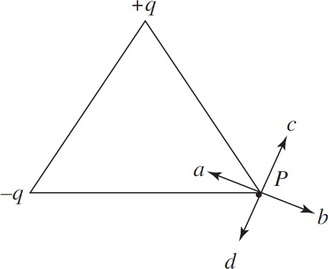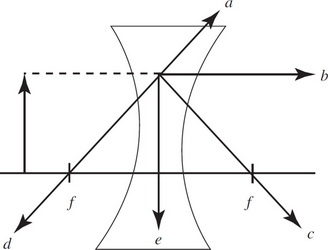# AP Physics 2 Practice Test 6

### Test Information10 questions18 minutes

1. Question below refers to the following figure:Two charges are placed as shown at the vertices of an equilateral triangle. What is the direction of the electric field at point P ?

2. Question below refers to the following figure:A hollow conducting sphere is placed around point P so that the center is at P. What happens to the electric field strength at point P ?

3. A machine shoots a proton, a neutron, or an electron into a magnetic field at various locations. The paths of two particles are shown above. Assume they are far enough apart so that they do not intersect and the magnetic field is going out of the page. What can you say about the paths that represent each particle?

4. Heat is added to a cylindrical aluminum rod of radius Q and length . The temperature difference between the two ends of the rod varies from 10°C to 20°C. What geometric factors will influence the rate heat is transferred along the rod?

5. A rigid, solid container of constant volume holds an ideal gas of volume v1 and temperature T1 and pressure P1. The temperature is increased in an isochoric process. Which of the following is NOT true?

6.In the figure above, a ray of light hits an object and travels parallel to the principal axis as shown by the dotted line. Which line shows the correct continuation of the ray after it hits the concave lens?

7. A gas undergoes an expansion-compression cycle. If, plotted on a P-V diagram, the cycle is counterclockwise and the work is 300 J in magnitude, what was the heat transfer during this cycle?

8. Ultraviolet light has a wavelength of about 6 × 10-8 m. What is the frequency of this light?

9. Question below refers to the following situation:

Photons are emitted only when incoming radiation of wavelengths less than 2 × 106 m illuminates a metallic surface.

If you double the wavelength, the number of photons emitted will

10. Question below refers to the following situation:

Photons are emitted only when incoming radiation of wavelengths less than 2 × 106 m illuminates a metallic surface.

The cutoff frequency is